# Transformations in Machine Learning

Take a journey through decades of transformations in Deep Learning.

·Published Jul 12, 2022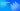On 8th September 2020, an article in the Guardian was written by a robot called GPT-3. They asked the robot to write an article about why humans should not be scared of robots and Artificial Intelligence. The human editors wrote the introduction for the article and instructed GPT-3 to generate the next possible sentences iteratively. Finally, the Guardian editors compiled those sentences into the article you can read here.

In this article, we will discuss the transformations in the field of Natural Language Processing that made it possible for a robot to write that is difficult to distinguish from human writing. We will begin our journey with Perceptrons, which laid the mathematical foundations of the Neural Networks.

Next, we will discuss convolutional neural networks (CNNs), which are state-of-the-art models for image classification, and long short-term memory networks (LSTMs) used by major speech engines. Then, we will look at Transformer-based models, which have set new benchmarks for natural language tasks. Finally, we will conclude with how GPT-3 combines previous benchmark models' learning and where it can take us in the future.

But before we start, let’s quickly discuss what GPT-3 is and how it works.

## What Is GPT-3?

Generative Pre-trained Transformer 3 (GPT-3) is a state-of-the-art language model with 175 billion trainable parameters built by OpenAI in 2020. It generally works by taking in an input text it uses to predict and generate relevant content. To give you a real-world example, we used the GPT-3 playground to explain what GPT-3 is by feeding it the following text:

“GPT-3 is a”

Here’s the output:

“GPT-3 is a natural language processing (NLP) model that uses deep learning to produce human-like text. It was created by OpenAI, an artificial intelligence research laboratory.”

Pretty cool, right?

GPT models generally predict the next word, which should follow the given input words and include it in the inputs for the next iteration. By iteratively generating words this way, the GPT models can construct coherent sentences that can be used for text completion tasks.

In March 2022, GPT-3 models became more powerful with the ability to suggest edits and inserts along with text completion. OpenAI is planning to launch the GPT-4 model soon, which is expected to have 100 trillion trainable parameters.

Now that you’re familiar with GPT-3 and how it works, let’s take a look at Perceptrons.

## Perceptrons: Learning from Numbers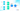A perceptron is a linear binary classifier algorithm and is the smallest indivisible building block of a Deep Neural Network (DNN). It is also referred to as an artificial neuron since it mimics the functionality of a biological neuron.

In the summer of 1969, the book titled “Perceptrons: An Introduction to Computational Geometry” was first published and dedicated to Frank Rosenblatt, who built the first Perceptron model in 1958. The book provided the core mathematical concepts of DNNs and defined the perceptron algorithm as a parallel algorithm capable of making decisions by linearly combining weighted inputs.

### How Perceptrons Work:

A Perceptron model has the following three components:

1. Bias (w0): A constant that shifts the decision boundary away from the origin, allowing perceptrons to return a non-zero output when all inputs are zero.
2. Weights (w1 and w2): Trainable parameters whose values are initialized randomly and adjusted during each training epoch in case of an error.
3. Activation Function (step function): Maps the weighted sum of inputs to the output as a boolean flag. The choice of the function allows us to configure the shape of the decision boundary.

To understand perceptrons, let’s consider the task of building a logical AND operator for two binary inputs: x1 and x2. The perceptron's output should be 1 only if both the inputs are 1. In case any of the inputs are 0, the perceptron should output 0. Since this is a linearly separable problem, a perceptron can solve it by learning optimal bias (w0) and weights (w1 and w2) representing the decision boundary y as: `w0 + w1*x1 + w2*x2`.

A step function is applied over the weighted sum of the inputs to generate the output of the perceptron as: `step(y) = {1 if (y > t) else 0}`, where `t` is the threshold. If the weighted sum is above the configured threshold only then the output is 1; otherwise, it is 0.

Perceptrons learn by trial and error. Assuming that the weights and threshold are initialized as 0.5, the perceptron gives correct results for the inputs `(0, 0)` and `(1, 1)`, but incorrect results for `(1, 0)` and `(0, 1)` as follows:

``````    Given inputs (0, 0) => y = (0.5 + 0.5*0 + 0.5* 0) = 0.5 & output = step(0.5) = 0 (correct)
Given inputs (0, 1) => y = (0.5 + 0.5*0 + 0.5* 1) = 1 & output = step(1) = 1 (incorrect)
Given inputs (1, 0) => y = (0.5 + 0.5*1 + 0.5* 0) = 1 & output = step(1) = 1 (incorrect)
Given inputs (1, 1) => y = (0.5 + 0.5*1 + 0.5* 1) = 1.5 & output = step(1.5) = 1 (correct)``````

The goal of the training process is to minimize the error (e), which is computed as the difference between the expected output (0) and the actual output (1). The error can be reduced by updating the weights for each incorrect prediction as: `wi = w1 + Lr*e`, where Lr is the learning rate. Considering a learning rate of 0.3, the weights are updated as: *wi = 0.5 + 0.3 (0 - 1) = 0.2**

In the second epoch, we notice that the perceptron is able to give correct classifications for all inputs as follows:

``````    Given inputs (0, 0) => y = (0.2 + 0.2*0 + 0.2* 0) = 0.2 & output = step(0.2) = 0 (correct)
Given inputs (0, 1) => y = (0.2 + 0.2*0 + 0.2* 1) = 0.4 & output = step(0.4) = 0 (correct)
Given inputs (1, 0) => y = (0.2 + 0.2*1 + 0.2* 0) = 0.4 & output = step(0.4) = 0 (correct)
Given inputs (1, 1) => y = (0.2 + 0.2*1 + 0.2* 1) = 0.6 & output = step(0.6) = 1 (correct)``````

​​The training ends when adjusting the weights no longer substantially reduces the error (that is, the model has converged to the minimum error). Since the error is zero for all inputs after the second epoch, we can say that the perceptron has learned to behave similarly to a Logical AND gate. Similarly, a perceptron can be trained for other linearly separable tasks (like OR gate), though it fails to converge for a non-linear task like XOR gate.

In the 1980s, it became possible to build more complex networks of perceptrons with the introduction of the backpropagation algorithm. An Artificial Neural Network (ANN) is a collection of neurons connected in a network, often aggregated as layers. FeedForward Neural Network is a special type of ANN in which there are no loops (that is, the information flows in a single direction). All layers between the input layer and output layer are called hidden layers.

A Multi-Layered Perceptron (MLP) is a special type of feedforward network which has at least one hidden layer, and each layer is fully connected (that is, each neuron connects to all the neurons in the subsequent layer). A backpropagation algorithm allows the propagation of error gradients from the output layers to the input layer via hidden layers. This allows Perceptrons to be extended to non-linear tasks. An MLP with five neurons can implement more complex functions such as the XOR gate. We can train extremely complex functions for high-dimensional data by increasing the number of neurons and layers.

### What GPT-3 Learned from Perceptrons

To implement the AND gate, we used a single perceptron with two trainable parameters (w1 and w2). In contrast, the GPT-3 is an implementation of a feedforward neural network with 175 billion trainable parameters. It stacks 96 neural layers on top of each other to be able to generate natural language text. Thus, it is vital to understand the working of a perceptron to dive deeper into the field of Deep Learning.

### Limitations of Perceptrons

The limitation of perceptrons (or any Deep Learning method) is that they can only take real numbers as input. Thus we need to convert the text, image, video, or speech data into numbers to use in the downstream neural networks. In subsequent sections, we will explore the common methods for processing images, speech, and text.

## CNNs: Learning from Images

A Convolutional Neural Network (CNN) is a specialized feedforward neural network that encodes an image or other high-dimensional data into a low-dimensional space which can be used for further classification tasks. CNNs – or ConvNets – convert a large image into a smaller one by convoluting an image's area into a single point.

CNNs were first introduced in the 1980s, but they became popular with the Lenet-5 model in 1998. Lenet-5 is a 5-layered CNN that identifies handwritten digits. It has three convolution layers connected by two pooling layers for reducing dimensionality. The subsequent layers are for classifying the convoluted image to one of the ten digits (0 to 9).

### How CNNs Work: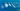A CNN model has the following three components:

1. Convolution Layer: Generates a low-dimensional feature map from the input image. It applies a trainable kernel or filter over a portion of the image and can extract low-level features like edges and color.

2. Pooling Layer: Further reduces the dimension of the feature map by taking either maximum or average of the feature value from a portion of the feature map. It allows us to extract the dominant features and suppress the noise in the input image.

3. Fully Connected Layer: Flattens the feature map and connects the CNN to the output layer or classification layer. It combines low-level and dominant features so downstream layers can learn to classify the image correctly.

To understand CNNs better, let’s consider the example of the Lenet-5 model, which has 60,000 trainable parameters. Given an 8-bit grayscale image of 32x32 pixels (px) as input, the model needs to classify it as a handwritten digit (0 to 9). The image can be represented as a matrix of size (32, 32); that is 1,024 floating-point numbers, each in the range of 0 to 256 on the grayscale.

The first convolution layer of Lenet-5 applies a 5x5 filter over the image to generate a feature map represented as a 28x28 matrix, where each point encodes the information from up to 25 neighboring pixels. Six such feature maps are generated in parallel and fed to the subsequent average pooling layer, which halves the size of the feature map. Thus, Lenet-5 generates six feature maps, each represented as a 14x14 matrix, making the output of the first two layers.

The second convolution layer applies 16 more 5x5 filters over the feature maps from the previous layer. This generates a feature map represented as a 10x10x16 matrix, which is halved by the average pooling layer to a 5x5x16 matrix. The third convolution layer applies 120 more 5x5 filters, each reducing the image to a single point. It generates a flat feature map consisting of 120 floating-point numbers. These 120 features extracted by convolution layers include the image’s features, like edges, colors, and shapes, invariant to image translation. Convolution allows us to recognize patterns in images similar to how the human brain would behave.

Finally, a fully connected layer consisting of 84 neurons connects the 120 features to the ten possible outputs (0 to 9). The model is trained in a supervised manner using the backpropagation algorithm.

Since the mid-2000s, Graphical Processing Units (GPU) have helped accelerate the development of more complex CNN architectures allowing the use of higher resolution images. In 2014, the Visual Geometric Group (VGG) of Oxford University released a VGG16 model pre-trained on 1.3 million images, each labeled across 1,000 classes. The pre-trained convolution layers of VGG16 can be used to train custom classification layers for various tasks, including facial recognition.

## What GPT-3 Learned from CNNs

Similar to CNNs, GPT-3 also separates the feature extraction and classification steps. This allows deep neural networks to be trained with limited amounts of training data using the transfer learning approach, which aims to re-use previously learned knowledge for a new task. The encodings from a pre-trained CNN or GPT model can be used for building custom models for a specific task. GPT-3 learns a 300-dimensional embedding for each word or token in its vocabulary of over 50 thousand tokens. These embeddings are fed to the input layers of the feedforward layer to generate an encoding that captures the global context. The output encodings can be further used to decode the next possible word for the given input sentence or similar natural language tasks.

### Limitations of CNNs

The limitation of CNN or any complex deep learning method is that it requires a large amount of data for training the neural network. More trainable parameters generally mean we need more training data. Overfitting with a large amount of training data becomes a common problem since selecting a representative sample from the entire population is challenging.

CNN cannot remember context from a sequence of inputs, which is important for speech recognition and natural language tasks. In subsequent sections, we shall explore how neural networks can remember context using memory cells and attention layers.

## LSTM: Learning from Speech

Long Short Term Memory (LSTM) cells are specialized neural networks to process a sequence of data (e.g., speech or video). It can memorize the previous inputs and utilize this memory to process subsequent inputs.

LSTM was first introduced in the 1990s, but the first successful application was developed in 2005 for speech recognition tasks. LSTM is a Recurrent Neural Network (RNN), which are networks capable of memorizing dependencies within data sequences, such as time series. Unlike feedforward networks, RNN has a loop connecting the output layer with the input layer.

### How LSTMs Work: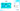An LSTM cell has the following important components:

1. Input Gate combines the current inputs with the previous hidden state, which is used to compute the predictions and update the memory in the cell state.

2. Forget Gate decides if the cell state needs to be reset. It combines the current inputs with the previous hidden state. It returns a value between 0 (completely forget) and 1 (completely remember), which is used to update the memory in the cell state.

3. Output Gate generates the next hidden state by combining the current inputs and the previous hidden state with the stored memory in the cell state. The output hidden state is also used for generating predictions.

4. Cell State is a memory block that stores the current state of the LSTM unit. The memory in the cell state is updated by combining the input gate and forget gate results along with the existing cell state. The updated cell state is used to compute the next hidden state.

To understand the working of LSTM, let’s consider the first successful application built using LSTM for speech recognition in 2005. Given an audio file with a sampling rate of 16 kHz, the task is to recognize the phonetics uttered in the audio file. The audio signal is represented as a time series of amplitudes of the audio wave, and we have 16K such values for each second in the range of 16-bits, i.e., 32K to +32K. The signal is broken into small time windows of 10 ms for extracting features from raw audio files. Then, Fourier Transform is applied over the signals in each window, and the result is converted to mel scale, which represents a frequency scale according to a human's hearing ability. Mel Scale has small intervals for low-frequency signals while large intervals for high-frequency signals. Finally, the frequency distribution is computed for 26 wavelengths from the normalized signal in mel scale. Thus for each time window of 10 ms, 26 features are extracted called Mel Frequency Cepstral Coefficients (MFCC).

The first successful implementation in 2005 for speech recognition has 200,000 trainable parameters. The input layer has 26 neurons to ingest a single window or frame in a single pass. It uses two hidden layers built with 140 LSTM cells each, one capturing the context in forward direction and the other backward. The output layer has 43 nodes, one for each possible phonetic. The LSTM cells can memorize the signals in the previous frames to predict the correct phonetics. The backpropagation algorithm is used to train the neural network.

In 2015, Google used LSTM for speech recognition on Google Voice. Similar implementations have been used for most digital assistants, including Siri and Alexa.

### What GPT-3 Learned from LSTM

Similar to LSTM, GPT-3 can utilize context over short and long distances. Instead of feeding one input at a time, GPT-3 can consume a long sequence of inputs at once and can combine contexts from all the inputs irrespective of the position of the sequence. First, the input sentence is split into words or tokens. It takes a maximum of 2048 words or tokens as inputs, each represented as a 300-dimensional vector. It combines each context to generate a single context vector that can be used to predict the next word in the sequence or other natural language tasks.

### Limitation of a LSTMs

The limitation of LSTMs or any deep learning model over sequential data is that they are very costly during inference regarding response time and resource usage. Since the next predictions depend on previous outputs of the model, it needs to run the model sequentially for each time window. This demands the usage of large machines, possibly with GPUs, to generate real-time predictions in production environments.

Another limitation of LSTMs is that the context propagates only in one direction. The only way to use future context is to add a parallel network of LSTMs that consumes inputs in reverse order. Both past and future contexts are important for solving natural language tasks. In the next section, we explore how transformers can be used to capture the context effectively from both directions.

## Transformer: Learning from Text

A transformer is a specialized feedforward neural network that encodes a sequence of inputs to a sequence of vectors by combining context from local and global patterns. The encodings can be decoded as a sequence of outputs or used for other downstream classification tasks.

Transformer architecture was first introduced in 2017 in the highly cited paper Attention is all you need, which used attention layers instead of recurrent or convolution layers for translating English text to the French language. Six attention layers were used to encode a sequence of natural language text, and another six attention layers were used for decoding the translated text. Thirty-six million sentence pairs for English to French were used for training these layers, comprising 32 thousand unique words.

### How Transformer Work: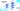Each attention layer in a transformer has the following three components:

1. Attention head combines the context from the local and global patterns by learning three matrices: Query, Key, and Value. Multi-headed attention mechanism allows the use of multiple attention heads working in parallel to capture different aspects of the natural language. The output of each self-attention head is computed by combining the dot products of each matrix with the given input sequence.

2. Feed Forward Network adds and normalizes the outputs from the multiple attention heads and connects them to the next attention layer.

3. Positional encoding is an additional input to capture the sequence's order of inputs. Since the whole sequence is fed into the network at once, the information about the order of inputs is lost. The positional information is encoded as a sine function of fixed frequency.

To understand the transformer, let us consider the task of translating English sentences to French Sentences using the original model with twelve attention layers, each having eight attention heads. Given an English sentence as input, it is split into a list of words or tokens. The transformer maintains a vocabulary of 32 thousand tokens and learns 512-dimensional embedding for each token. The embeddings of the input tokens are fed into the first attention layer, which encodes the context and forwards it to the next layers. The seventh attention layer starts decoding the next possible word using the encodings from the previous layers. The output of the twelfth attention layer is fed into a classification layer having 32 thousand possible outcomes, each representing a unique token from the vocabulary.

The output token is appended to the input sequence along with a special separator token and fed again to generate the next word. The encoder layers only use the English sentence, but the decoder layers use both English encodings and generated french tokens. Iteratively, transformers generate one french token at a time combined to return the translated French Sentence.

Bi-directional Encoder-Decoder Representations from Transformers (BERT) is a popular implementation of the Transformer Architecture, having 110 million trainable parameters. A pre-trained model was released in 2019, which was trained on 3.2 billion words from books and Wikipedia. Using a transfer-learning approach, the base BERT model can be extended to many natural language tasks, like sentiment analysis, question-answering, and more. An extra classification layer can be trained using labeled training data for fine-tuning the pre-trained BERT model.

The large size of Transformer models becomes a challenge to use in real-time applications. To counter this, smaller transformer models were trained using the distillation process called DistillBERT with only six attention layers. During distillation, a smaller student model tries to imitate the results from the larger teacher model. DistillBERT is 40% smaller and 60% faster than the base BERT model making it usable for real-time applications.

### What GPT-3 learned from Transformers

GPT-3 is the state-of-the-art transformer model for generating text sequences using ninety-six attention layers, each having 96 attention heads. It uses few-shot learning to adapt to different tasks during the inference. The first input sentence is the instruction of the task along with a few examples, and the second input sentence is the initial words for which output needs to be generated. Similarly, in one-shot learning, only one example is given along with the instruction and in zero-shot learning, only instruction is given without any examples. Fine-tuning language models often require a large set of labeled data for a specific task, but few-shot learning allows for learning new tasks where limited labeled data is available.

### Limitation of a Transformers

The limitation of Transformer or any large deep learning algorithm is that they behave like a black box, and the results are hard to explain. Sometimes the outputs might be incoherent and gibberish. It is hard to prove that the models learn a language and do not just memorize the training data. Also, costly inference and the requirement of large training data hinder their wide-scale applications.

Transformer models often exhibit bias towards gender, race, and religion. This bias is the vast internet data from which they learn. Often public data used for training reflect the biases inherent in human society, and models tend to learn those biases and build an understanding of the natural language.

The Transformers were initially designed for language translation tasks but have been adapted for many tasks, including image classification and speech recognition. Lastly, we conclude with the different use cases for transformer-based models.

## Wrapping it all Together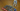We saw the journey of the transformations in the field of deep learning throughout the decades. Coming back to the article published in Guardian is an exemplary demonstration of the capabilities of modern transformer-based models like GPT-3. The editors used the zero-shot learning approach by providing only instruction and initial sentences. The instructions given to GPT-3 were “I am not a human. I am Artificial Intelligence. Many people think I am a threat to humanity. Stephen Hawking has warned that AI could “spell the end of the human race.” Using these two inputs, eight articles were generated using a pre-trained GPT-3 model. Finally, the editors combined those eight into the one that got published.

Overall, most modern deep learning methods attempt to generate data embedding, which can be used for further downstream tasks. Though convolution and recurrent layers fail to capture distant context, attention layers of transformers are very efficient at combining local and global contexts.

Transformers have been used in combination with other neural networks for a wide range of tasks. For speech recognition tasks, wave2vec is the state-of-the-art model which combines CNN and Transformers. CoCa is the state-of-the-art model for image classification tasks built using visual transformers. Recently, Megatron has beaten GPT-3 as the state-of-the-art model for language modeling tasks making improvements to the existing transformer implementations.

### Other use cases of GPT-3

Along with writing articles for The Guardian, GPT-3 has found many other interesting use cases. GitHub Copilot can generate code based on natural language instructions using a descendant of the GPT-3 model called OpenAI Codex. DallE is a 12 billion parameter version of GPT-3 trained to generate images from textual descriptions, while OpenAI Clip is another version trained to generate textual descriptions from images. For generating music, the Jukebox model was built using CNNs and Transformers. GPT-3 is also used in the text-based adventure game called AI Dungeon.